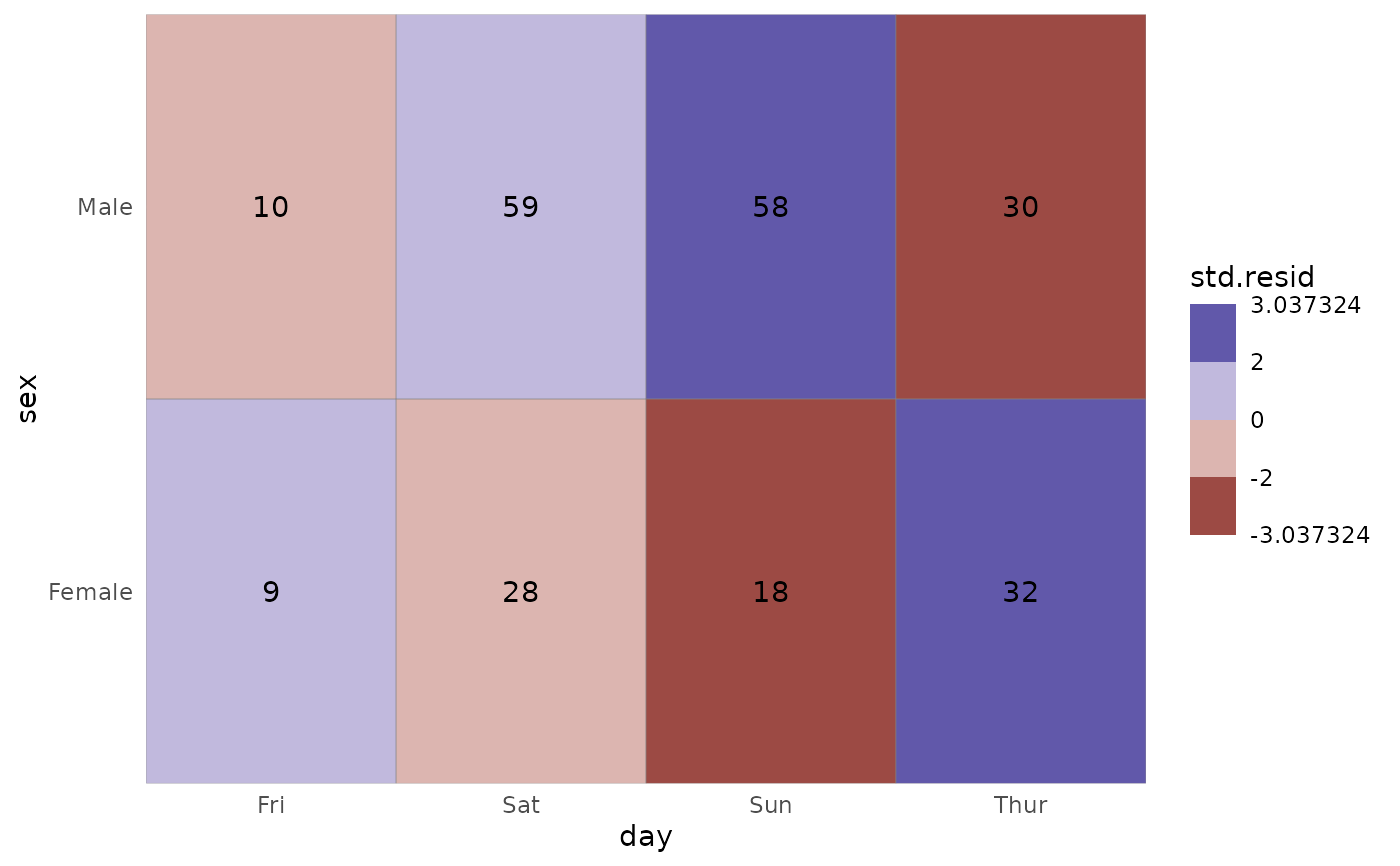ggally_crosstable is a variation of ggally_table with few modifications: (i) table cells are drawn; (ii) x and y axis are not expanded (and therefore are not aligned with other ggally_* plots); (iii) content and fill of cells can be easily controlled with dedicated arguments.

ggally_crosstable(
data,
mapping,
cells = c("observed", "prop", "row.prop", "col.prop", "expected", "resid",
"std.resid"),
fill = c("none", "std.resid", "resid"),
...,
geom_tile_args = list(colour = "grey50")
)

## Arguments

data data set using aesthetics being used Which statistic should be displayed in table cells? Which statistic should be used for filling table cells? other arguments passed to geom_text(...) other arguments passed to geom_tile(...)

## Examples

# Small function to display plots only if it's interactive
p_ <- GGally::print_if_interactive

data(tips, package = "reshape")

# differences with ggally_table()
p_(ggally_table(tips, mapping = aes(x = day, y = time)))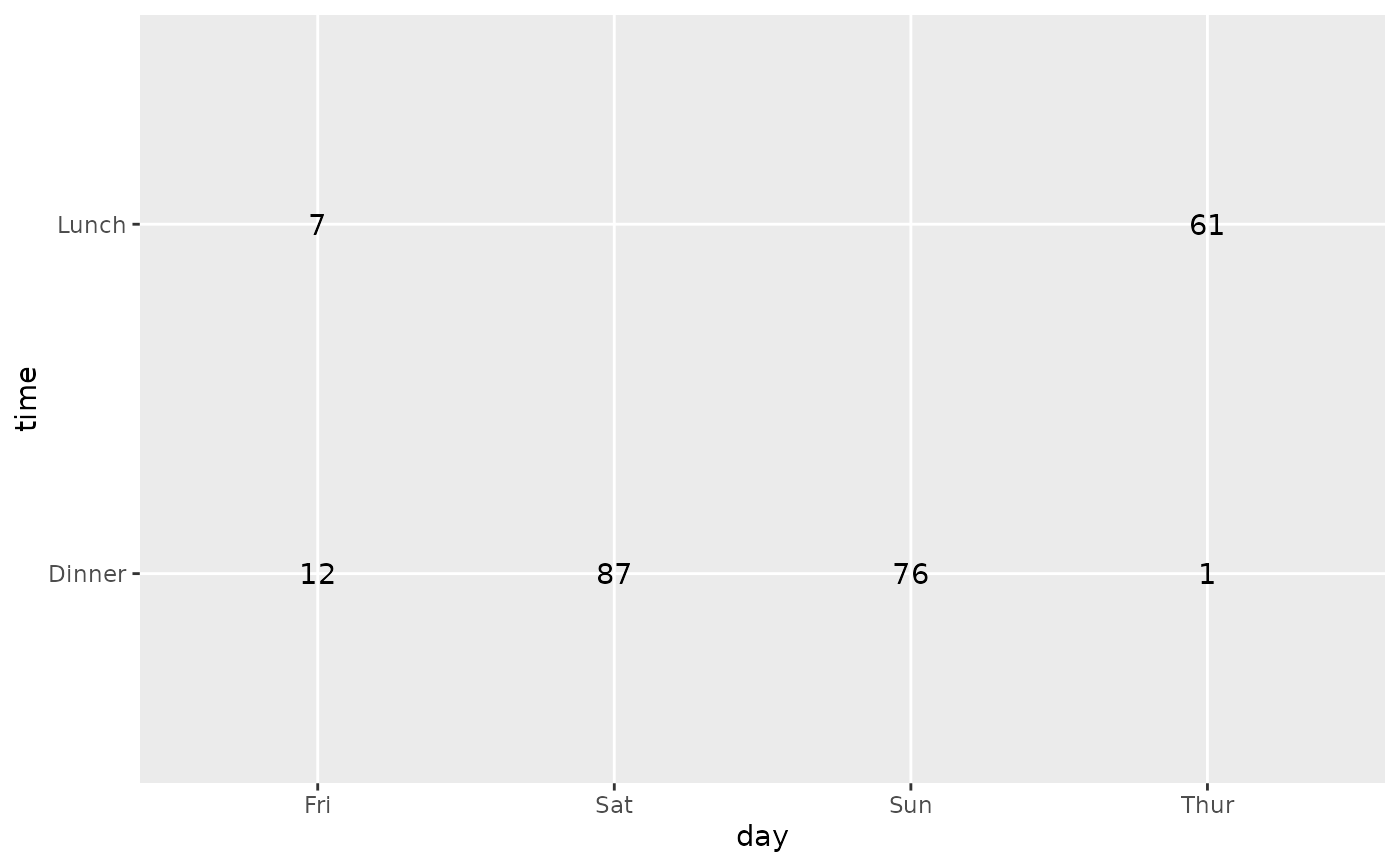p_(ggally_crosstable(tips, mapping = aes(x = day, y = time)))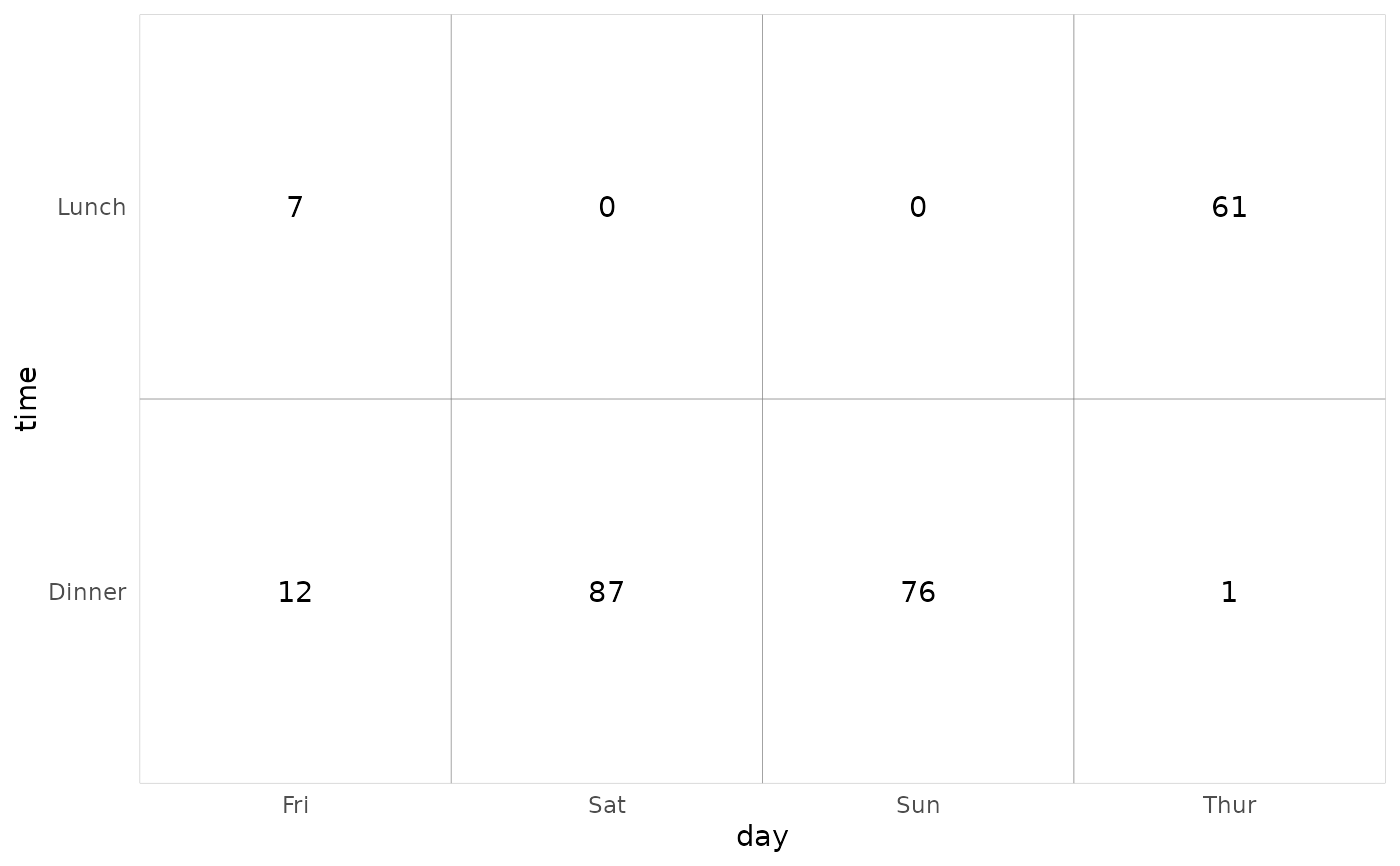# display column proportions
p_(ggally_crosstable(tips, mapping = aes(x = day, y = sex), cells = "col.prop"))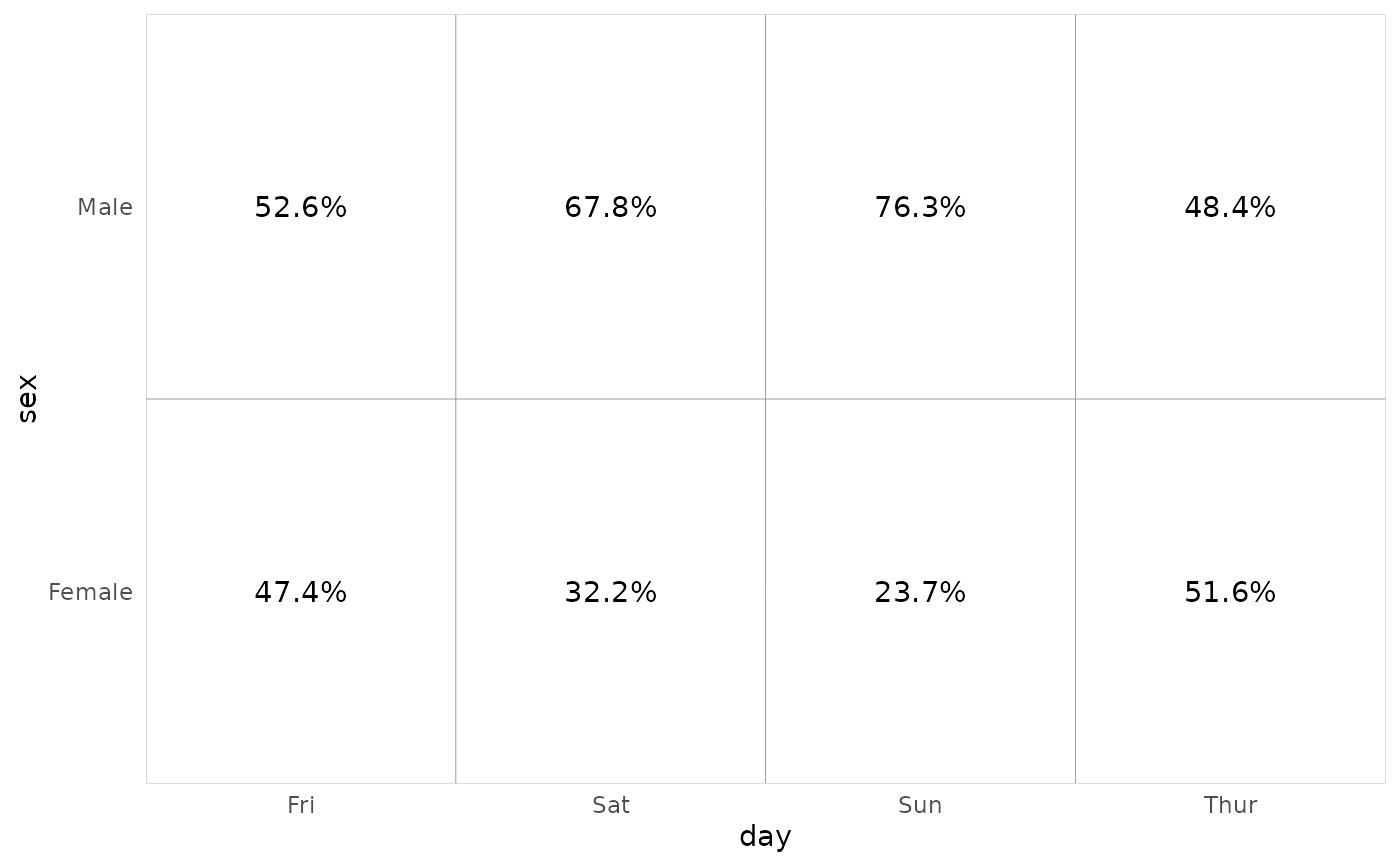# display row proportions
p_(ggally_crosstable(tips, mapping = aes(x = day, y = sex), cells = "row.prop"))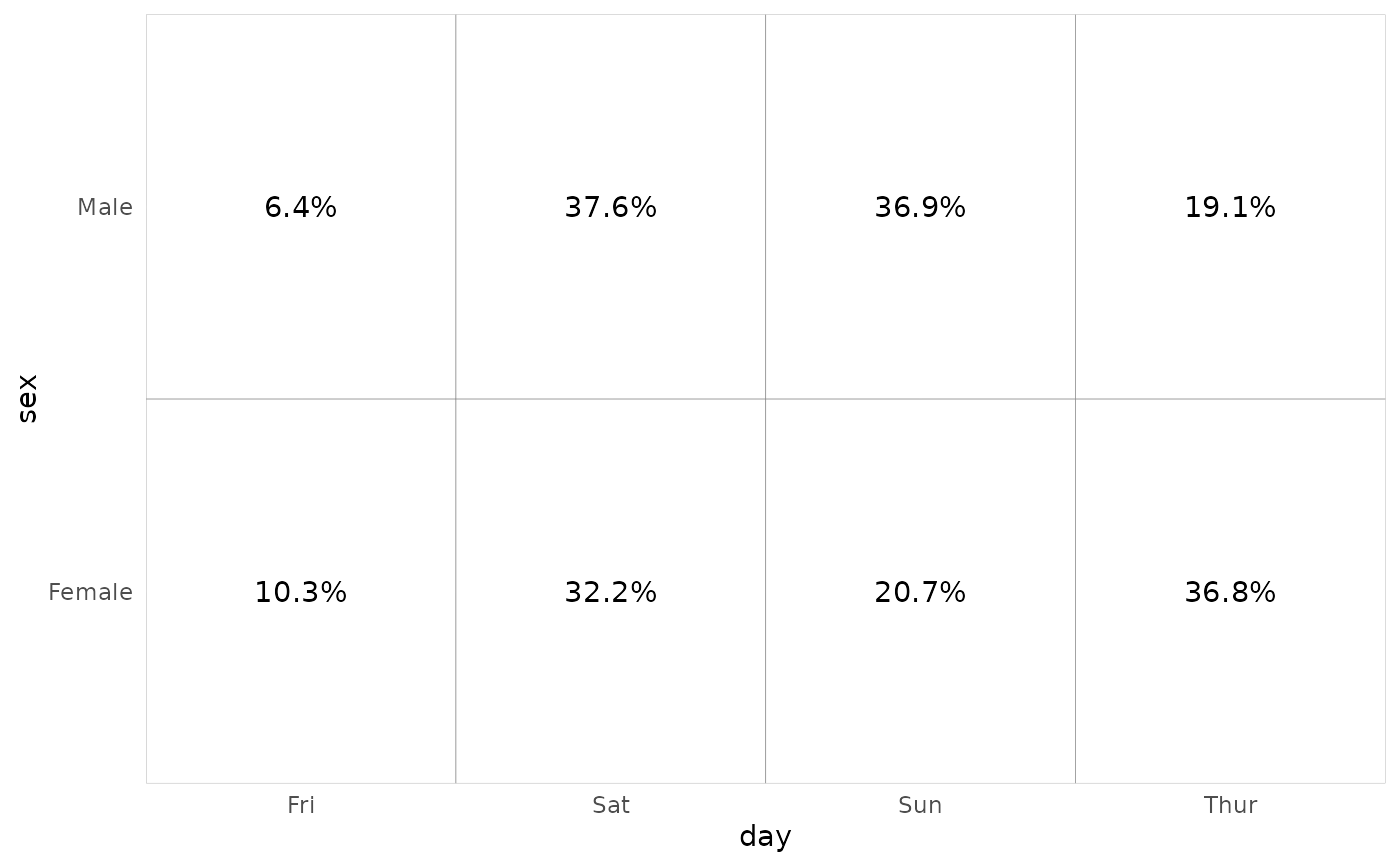# change size of text
p_(ggally_crosstable(tips, mapping = aes(x = day, y = sex), size = 8))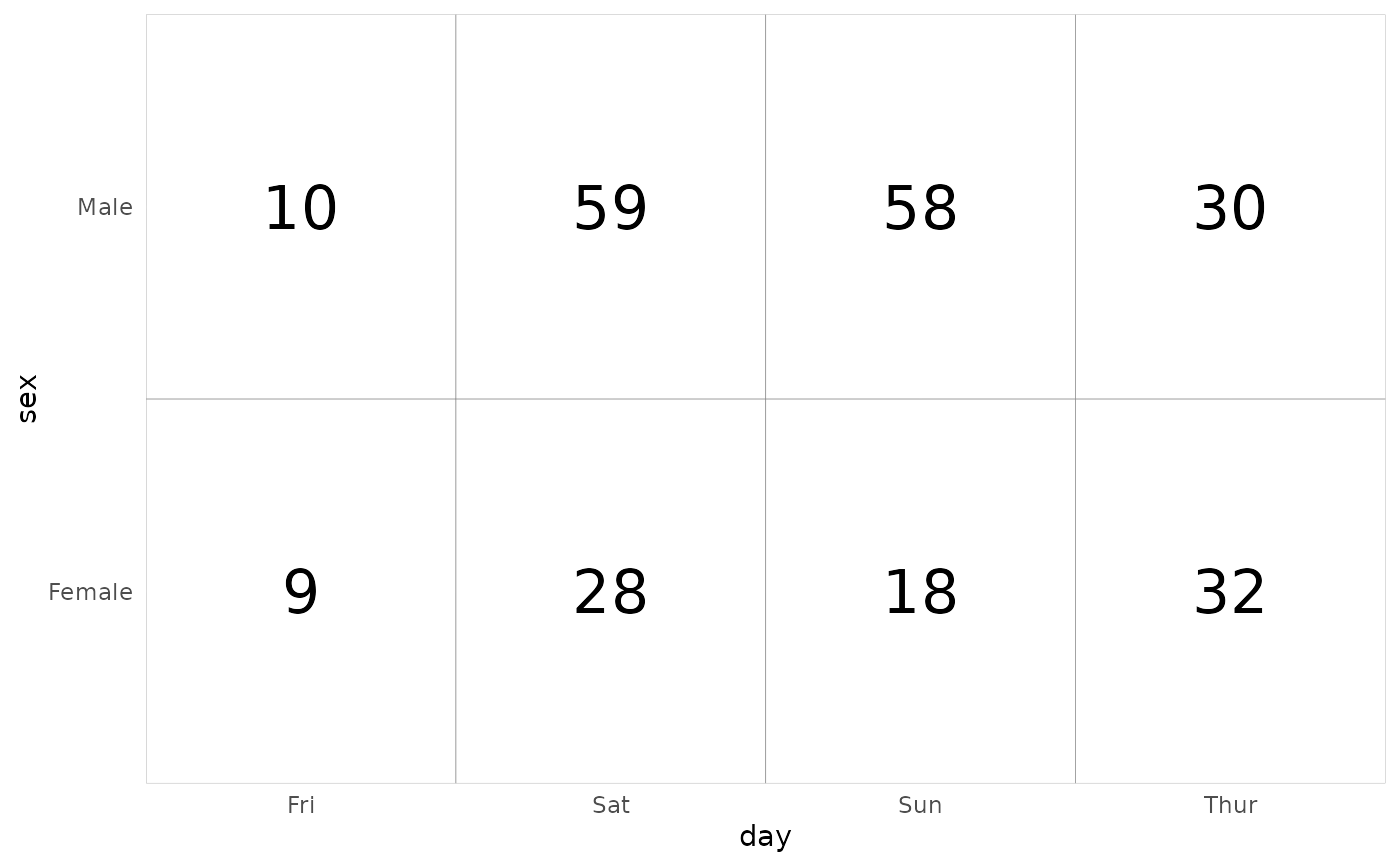# fill cells with standardized residuals
p_(ggally_crosstable(tips, mapping = aes(x = day, y = sex), fill = "std.resid"))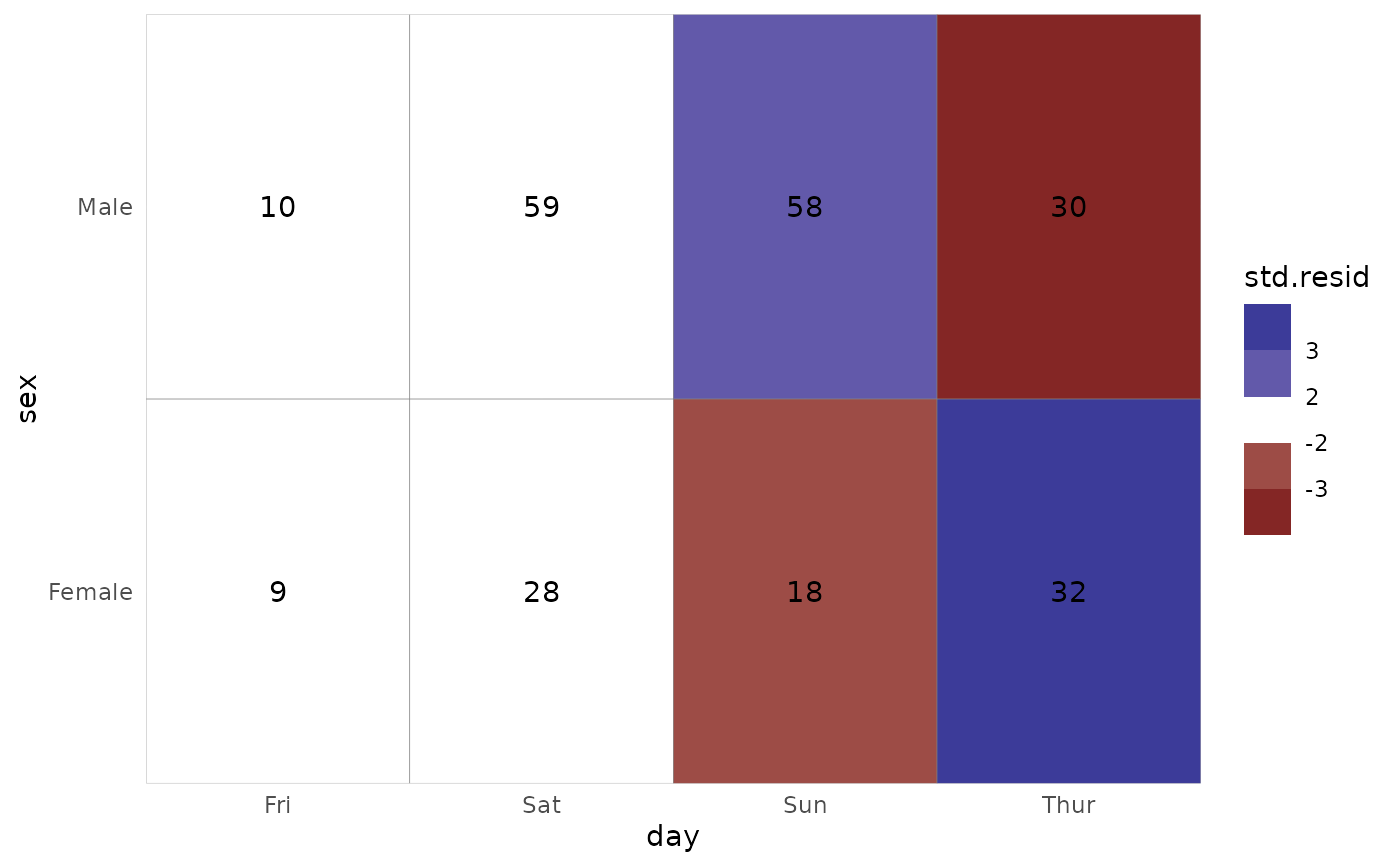# change scale for fill
p_(ggally_crosstable(tips, mapping = aes(x = day, y = sex), fill = "std.resid") +
scale_fill_steps2(breaks = c(-2, 0, 2), show.limits = TRUE))
#> Scale for 'fill' is already present. Adding another scale for 'fill', which
#> will replace the existing scale.